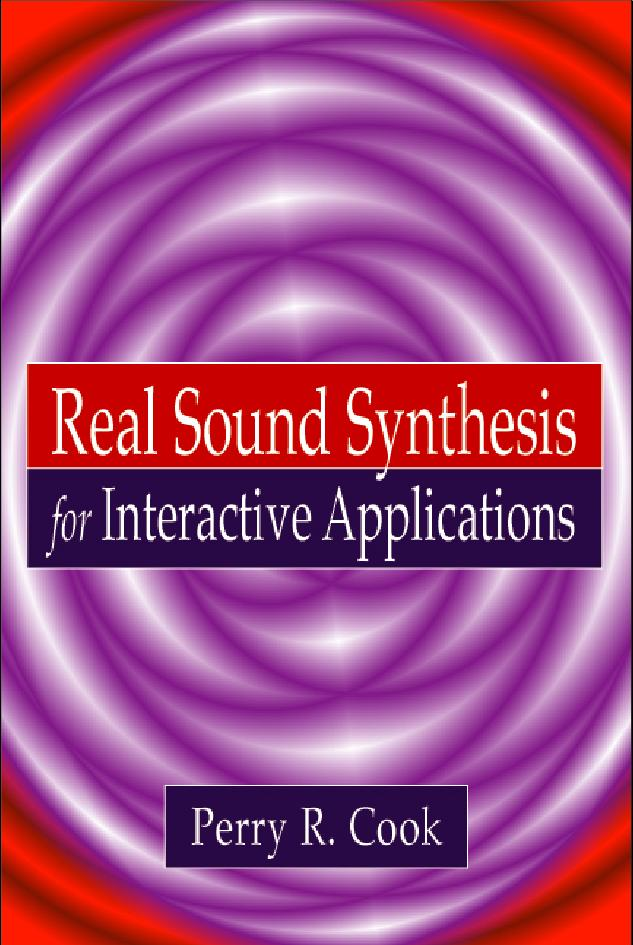Real Sound Synthesis for Interactive Applications by Perry R. Cook Errata Back to Main Book Page Details: Price: \$39    Year: 2002 250 page book with CD. ISBN: 1-56881-168-3

STK Examples, Appendix E
"syntmono" should be "demo"
There is now also a "syntpoly" (multi voice synthesis),
and lots of other new things in this new release.

Page 142
Equation 12.7 should be Labeled Equation 12.8

Equation numbers in Appendix B
last sentence, pg. 222 should read:
"Noting that Equations B.6 and B.7 are both..."

Page 5, 2nd Equation
The constant C at the end is equal to 10 log_10(12) = 10.8
but we drop it because it becomes less and less important
for increasing number of bits.
Our interest is the increase in SNR with the addition of
each bit, which is about 6 dB per bit.

Page 31, Section 3.9, Last Paragraph
The gains here are wrong. oops.
Gain for r=0.99 at SRATE/2 is actually 0.005, or -46dB.
In doing this section, I originally derived it using r=0.99998,
but decided to change it later, and didn't get the change folded in.

Page 214, Section A.3
The summation upper limits in Equations A.7 and A.8 should be "n"
rather than P-M-1. This is because of the causality relationship
(h(i) and x(i) are zero for any negative i), and the original
convolution summation given in Equation A.6 (if i exceeds n,
h will be zero because it has a negative index). Carrying that
idea forward will cause i to range from 0 to n, and also k
after the substitution. The good news of course is that
convolution is commutative, and can be done by DFT.

Page 54, Equation 5.7
f(n) should be italic f(n)

Page 54, Equation 5.8
Fb(n) and Fc(n) should be Fb(m) and Fc(m)

Page 55, Section 5.4 Those three orthogonality conditions are true for p, q, < N/2
The non-zero sum result, when p=q, is N/2